Students can download Maths Chapter 5 Coordinate Geometry Additional Questions and Answers, Notes, Samacheer Kalvi 9th Maths Guide Pdf helps you to revise the complete Tamilnadu State Board New Syllabus, helps students complete homework assignments and to score high marks in board exams.

## Tamilnadu Samacheer Kalvi 9th Maths Solutions Chapter 5 Coordinate Geometry Additional Questions

I. Multiple Choice Questions.

Question 1.
On which quadrant does the point (- 4, 3) lie?
(a) I
(b) II
(c) III
(d) IV
Solution:
(b) II

Question 2.
The point whose abscissa is 5 and lies on the x-axis is …….
(a) (-5, 0)
(b) (5, 5)
(c) (0, 5)
(d) (5, 0)
Solution:
(d) (5, 0)Question 3.
A point which lies in the III quadrant is ……..
(a) (5, 4)
(b) (5, -4)
(c) (-5, -4)
(d) (-5, 4)
Solution:
(c) (-5, -4)

Question 4.
A point on the y-axis is ……..
(a) (1, 1)
(b) (6, 0)
(c) (0, 6)
(d) (-1, -1)
Solution:
(c) (0, 6)

Question 5.
The distance between the points (4, -1) and the origin is ……..
(a) $$\sqrt{24}$$
(b) $$\sqrt{37}$$
(c) $$\sqrt{26}$$
(d) $$\sqrt{17}$$
Solution:
(d) $$\sqrt{17}$$

Question 6.
The distance between the points (-1, 2) and (3, 2) is ……..
(a) $$\sqrt{14}$$
(b) $$\sqrt{15}$$
(c) 4
(d) 0
Solution:
(c) 4Question 7.
The centre of a circle is (0, 0). One end point of a diameter is (5, -1), then the radius is …….
(a) $$\sqrt{24}$$
(b) $$\sqrt{37}$$
(c) $$\sqrt{26}$$
(d) $$\sqrt{17}$$
Solution:
(c) $$\sqrt{26}$$

Question 8.
The point (0, -3) lies on
(a) + ve x-axis
(b) + ve y-axis
(c) – ve x-axis
(d) – ve y-axis
Solution:
(d) – ve y-axis

Question 9.
The point which is on y-axis with ordinate -5 is ……..
(a) (0, -5)
(b) (-5, 0)
(c) (5, 0)
(d) (0, 5)
Solution:
(a) (0, -5)

Question 10.
The diagonal of a square formed by the points (1, 0), (0, 1), (-1, 0) and (0, -1) is …….
(a) 2
(b) 4
(c) √2
(d) 8
Solution:
(a) 2Question 11.
The distance between the points (-2, 2) and (3, 2) is ……..
(a) 10 units
(b) 5 units
(c) 5√3 units
(d) 20 units
Solution:
(b) 5 units

Question 12.
The midpoint of the line joining the points (1, -1) and (-5, 3) is ……..
(a) (2, 1)
(b) (2, -1)
(c) (-2, -1)
(d) (-2, 1)
Solution:
(d) (-2, 1)

Question 13.
If the centroid of a triangle is at (1, 3) and two of its vertices are (-7, 6) and (8, 5) then the third vertex is ……..
(a) (-2, 2)
(b) (2, -2)
(c) (-2, -2)
(d) (2, 2)
Solution:
(b) (2, -2)

Question 14.
The ratio in which the X-axis divides the line segment joining the points (6, 4) and (1, -7) is ……..
(a) 1 : 2
(b) 2 : 3
(c) 4 : 7
(d) 7 : 4
Solution:
(c) 4 : 7Question 15.
The centroid of a triangle (3, -5), (-7, 4) and (10, -2) is …….
(a) (2, -1)
(b) (2, 1)
(c) (-2, 1)
(d) (1, -2)
Solution:
(a) (2, -1)

Question 1.
Show that the given points (1, 1), (5, 4), (-2, 5) are the vertices of an isosceles right angled triangle.
Solution:
Let A (1, 1), B (5, 4) and G (-2, 5)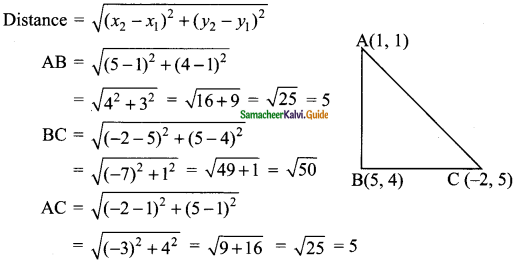AB = 5, AC = 5
∴ ABC is an isosceles triangle …….. (1)
BC² = AB² + AC²
50 = 25 + 25 ⇒ 50 = 50
∴ ∠A = 90° ……… (2)
From (1) and (2) we get ABC is an isosceles right angle triangle.

Question 2.
Show that the point (3, -2), (3, 2), (-1, 2) and (-1, -2) taken in order are the vertices of a square.
Solution: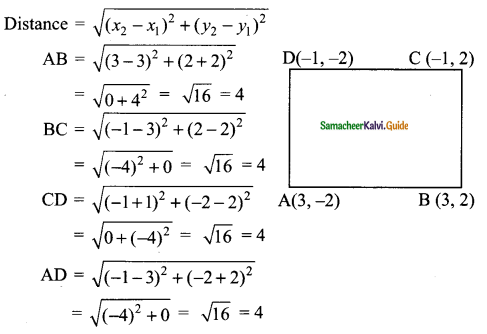= $$\sqrt{16}$$
= 4
AB = BC = CD = DA = 4. All the four sides are equal.
∴ ABCD is a Rhombus ……..(1)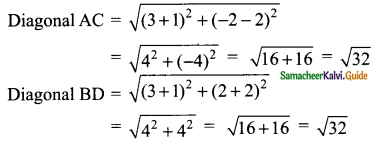Diagonal AC = Diagonal BD = $$\sqrt{32}$$ ……..(2)
From (1) and (2) we get ABCD is a square.Question 3.
Show that the point A (3, 7) B (6, 5) and C (15, -1) are collinear.
Solution: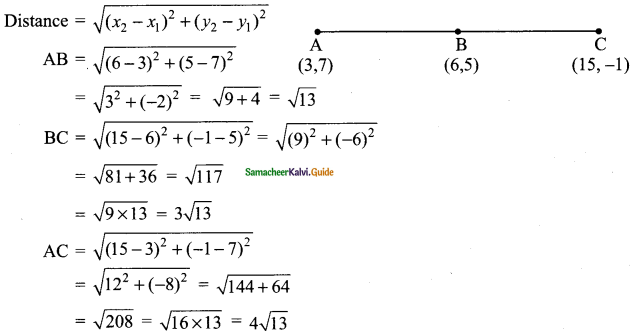AB + BC = AC ⇒ $$\sqrt{13}$$ + 3$$\sqrt{13}$$ = 4$$\sqrt{13}$$
∴ The points A, B, C are collinear.

Question 4.
Find the type of triangle formed by (-1, -1), (1, 1) and (-√, √3)
Solution:
Let the point A (-1, -1), B (1, 1) and C (-√3, √3)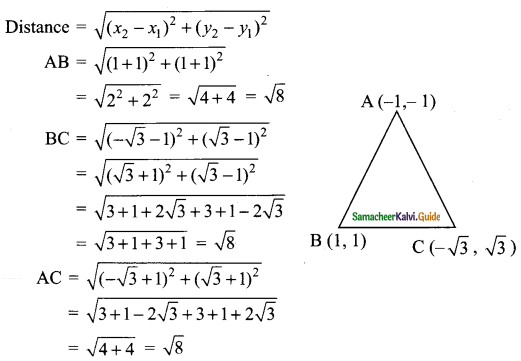AB = BC = AC = √8
∴ ABC is an equilateral triangle.

Question 5.
Find x such that PQ = QR where P(6, -1) Q(1, 3) and R(x, 8) respectively.
Solution: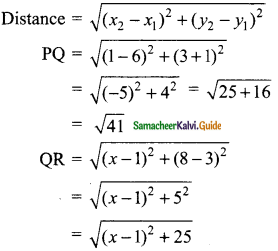But PQ = QR
$$\sqrt{(x-1)^{2}+25}$$ = $$\sqrt{41}$$
Squaring on both sides
(x – 1)² + 25 = 41
(x – 1)² = 41 – 25 = 16
x – 1 = $$\sqrt{16}$$ = ± 4
x – 1 = 4 (or) x – 1 = – 4
x = 5 (or) x = -4 + 1 = -3
The value of x = 5 or – 3Question 6.
Find the coordinate of the point of trisection of the line segment joining (4, -1) and
Solution:
Let A (4, -1) and B (-2, -3) are the given points
Let P (a, b) and Q (c, d) be the points of trisection of AB.
∴ AP = PQ = QB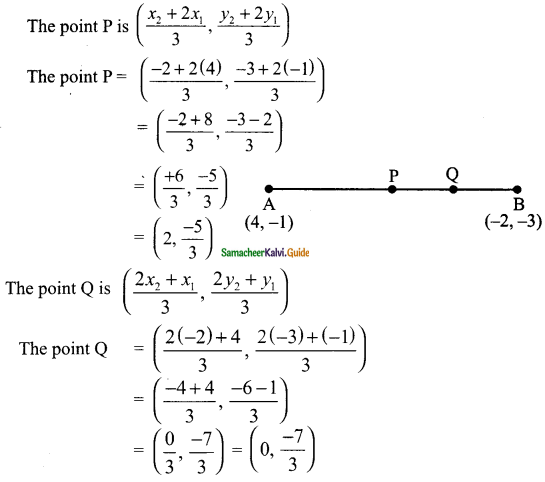The required coordinate P is (2, –$$\frac{5}{3}$$) and Q is (0, –$$\frac{7}{3}$$)

Question 7.
Find the ratio in which the line segment joining the points (-3, 10) and (6, -8) is divided by (-1, 6).
Solution:
Given points are A(-3, 10), B(6, -8) and P(-1, 6)
divides AB internally in the ratio m : n
By section formula.
A line divides internally in the ratio m : n the point P =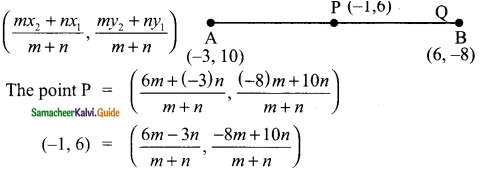∴ $$\frac{6m-3n}{m+n}$$ = -1
6m – 3n = -m – n
6m + m = 3n – n
7m = 2n ⇒ $$\frac{m}{n}$$ = $$\frac{2}{7}$$
∴ m : n = 2 : 7
and
$$\frac{-8m+10n}{m+n}$$ = 6
-8m + 10n = 6m + 6n
-8m – 6m = 6n – 10n
14m = 4n
∴ $$\frac{m}{n}$$ = $$\frac{14}{4}$$ = $$\frac{2}{7}$$
Hence P divides AB internally in the ratio 2 : 7Question 8.
If (1, 2) (4, y), (x, 6) and (3, 5) are the vertices of a parallelogram taken in order, find “x” and “y”.
Solution:
Let A(1, 2), B(4, y), C(x, 6) and D(3, 5)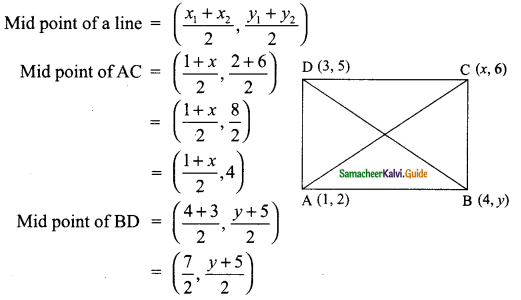Since ABCD is a parallelogram the diagonal bisect each other
Mid point of AC = Mid point of BD
($$\frac{1+x}{2}$$, 4) = ($$\frac{7}{2}$$, $$\frac{y+5}{2}$$)
$$\frac{1+x}{2}$$ = $$\frac{7}{2}$$
1 + x = 7
x = 7 – 1
= 6
and
$$\frac{y+5}{2}$$ = 4
y + 5 = 8
y = 8 – 5
= 3
∴ The value of x = 6 and y = 3